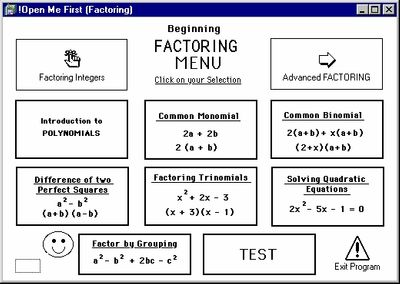Tutorial math and reading software for elementary and secondary arithmetic, basic math, algebra, geometry, precalculus plus GED, ABE, and CLEP preparation for elementary school, high school, college, adult education, and homeschool students.

# Program 2: "Factoring Polynomials"Item# FACTOR
\$49.00
Media Type:

## Product Description

[Click on sample screen to enlarge.]

From this program the student will learn factoring topics from both the first and second year algebra curriculum.

Factoring Integers is reviewed followed by an Introduction to Polynomials which includes instruction on monomials, binomials, and trinomials (adding, subtracting, and multiplying).

The First Year Algebra MENU continues with Factoring a Common Monomial, Factoring a Common Binomial, Factoring the Difference of Two Perfect Squares, Factoring Trinomials, Factoring by Grouping and Solving Quadratics.

The Second Year Algebra MENU leads the student through: Long Division, Synthetic Division, The Remainder Theorem, The Factor Theorem, Factoring Odd Power Binomials and concluding with a section on Graphing Techniques with and without a graphing calculator.